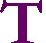S k i l l i n A R I T H M E T I CThe science of numbers and the art of calculation depend upon pure intelligence in the attainment of pure truth.

Indeed, it most certainly does that.

Have you noticed, too, how people with a talent for calculation are generally quick at every other kind of knowledge; and how training in it makes a slow mind quicker than it would otherwise have been?

I have.

Also, it would not be easy to find many branches of study that require more effort from the learner. For all these reasons we cannot do without this form of training.

I agree.

Plato, The Republic, Book VIIHE CALCULATOR has changed the face of arithmetic. What can we teach, then, that the student will actually find useful? What deserves to be called educational?

Our answer is to emphasize problems that should not require a calculator—or even pencil and paper.  For arithmetic is, and always has been, a spoken skill, based on knowing the addition and multiplication tables. To add something as simple as 28 + 6, one can simply say, "28 plus 2 is 30, plus 4 is 34."

How much is 50%, or Half, of 308?  "Half of 300 is 150.  Half of 8 is 4. Therefore Half of 308 is 154."

Those should not be written problems. And they certainly should not require a calculator.

The calculator has in fact freed arithmetic to resume its true nature, which is the art of counting.

Most of us however have grown up thinking we're supposed to do arithmetic with pencil and paper—which is itself a calculator. Those written methods give answers and so we are relieved of understanding. The very names—addition, subtraction, multiplication, division—have become the names of written methods.  To "subtract" 75 from 102 has come to mean: Write 75 under 102, draw a line,and do the method.

But the calculator has changed all that. Therefore we can now do more than teach those written rules. They will be found here, but my purpose is to rescue arithmetic from much of their crippling effect.

(To find the difference between 75 and 102: What number must we add to 75 to get 102?  "75 plus 25 is 100, plus 2 is 102.  27.")

I say in fact that we're supposed to do arithmetic mentally. It is that which distinguishes arithmetic from algebra, which is a written skill. We may use a calculator, electronic or written, when mental calculation is too difficult. But the teaching of arithmetic can now encourage number-sense—counting!—which is natural to the human mind. What is more, it strengthens the power of understanding.

Understanding that a certain number is ONE MORE than another.

Understanding that in SUBTRACTION, we must find what number to add.

Understanding that MULTIPLICATION by a whole number is repeated addition—even multiplication of a fraction.

Understanding that in DIVISON, we must find how many times
one number is contained in another.

Understanding that PERCENTper centum—means how many
for each 100.

And understanding PARTS.  For a unique feature of these pages is the meaning of aliquot parts: half of a number, a third, a fourth, a fifth—before they become names of fractions. Those names of the parts belong to language itself. Mathematics does not own them. In the sentences "5 is half of 10,"  "A quarter of the class got B," the wordshalf and quarter are not the fractions ½ and ¼.  The fraction ¼ is a number we need for measuring, it is one quarter or the fourth part of 1 on the number line.  Obviously, then, the student must first understand "one quarter." Confusion arises because in English (not every language), the proper fractions have the same names as the parts.  In fact, what is usually taught as fractions—"¼ means 1 out of 4"—is actually parts taught with fractional symbols.

But parts are more effectively taught verbally. They are the best preparation for fractions and percent.  In fact, many problems traditionally taught with fractions can now be understood directly. 5 people are what percent of 20 people? Since 5 is one quarter of 20, then 5 is 25% of 20. Why does 25% mean one quarter? Because 25 is one quarter of 100.

No fractions.

These pages, then, present arithmetic as its own discipline; not as a stepping-stone to algebra. This is not "pre-algebra." Algebra, which will be useful only to certain students, is the written manipulation of symbols. But arithmetic is not merely a relationship between symbols, for the words have meaning and therefore truth. "Three 4's are equal to 12." Properly taught, arithmetic is the most educational subject. When the algebra teacher gives the rule for dividing negative numbers, or the chemistry teacher asserts that a hydrogen atom has one proton, the student must accept it on authority. But the arithmetic student can see a fact itself. Five is half of ten. Whoever understands the meaning of those words can decide directly whether or not it is true.  It is not a question of authority or belief. That is an educational experience.